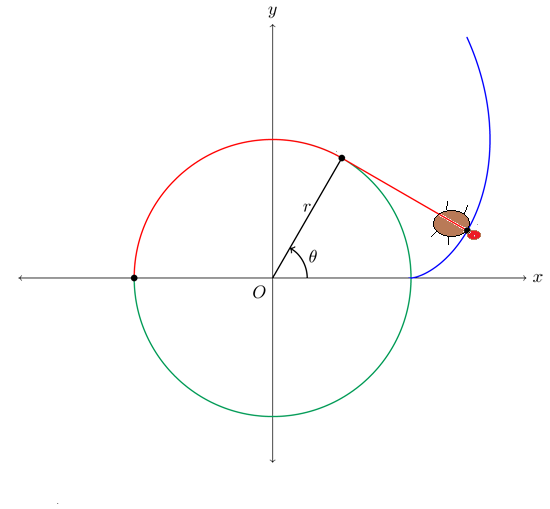# Involute of a circle by a sheep

Calculus Level 5A sheep is tied in a silo of radius $r$, by a rope long enough to reach the opposite end of the silo(see figure above). The total area available for the sheep to graze can be written as,

$\large\frac{p\pi^{3}r^{2}}{q},$

where $p$ and $q$ are co-prime positive integers. What is the value of $p+q$?

Note: The involute (of a circle), is the locus of points traced out by a taut string.
I found this problem in a book, and i thought i would share it.

×

Problem Loading...

Note Loading...

Set Loading...